Search
Document Center

# SELECT

Last Updated: Jun 18, 2021

The syntax of SELECT statements is complex. This topic describes the syntax of simple SELECT statements and the SELECT statements that contain set operators.

## SIMPLE SELECT

### Description

You can execute a simple SELECT statement to query table data.

### Syntax

``````simple_select:
SELECT [/*+ hint statement */] [ALL | DISTINCT | UNIQUE | SQL_CALC_FOUND_ROWS]
select_expr_list FROM from_list [WHERE condition]
[GROUP BY group_expression_list [WITH ROLLUP] [HAVING condition]]
[ORDER BY order_expression_list]
[limit_clause]
[FOR UPDATE]

select_expr:
table_name.*
| table_alias_name.*
| expr [[AS] column_alias_name]

from_list:
table_reference [, table_reference ...]

table_reference:
simple_table
| joined_table

simple_table:
table_factor [partition_option] [[AS] table_alias_name]
| (select_stmt) [AS] table_alias_name
| (table_reference_list)

joined_table:
table_reference [INNER] JOIN simple_table [join_condition]
| table_reference outer_join_type JOIN simple_table join_condition

partition_option:
PARTITION (partition_name_list)

partition_name_list:
partition_name [, partition_name ...]

outer_join_type:
{LEFT | RIGHT | FULL} [OUTER]

join_condition:
ON expression

condition:
expression

group_expression_list:
group_expression [, group_expression ...]

group_expression:
expression [ASC | DESC]

order_expression_list:
order_expression [, order_expression ...]

order_expression:
expression [ASC | DESC]

limit_clause:
LIMIT {[offset,] row_count |row_count OFFSET offset}``````

### Parameters

Parameter

Description

ALL | DISTINCT | UNIQUE | SQL_CALC_FOUND_ROWS

Specifies whether to return distinct table rows. A database table may contain duplicate values.

• If you add DISTINCT to the statement, only distinct rows are returned in the query results.

• If you add ALL to the statement, all the matched rows are returned.

• If you add SQL_CALC_FOUND_ROWS to the statement, only the number of rows is returned.

• If you do not add ALL, DISTINCT, UNIQUE, or SQL_CALC_FOUND_ROWS to the statement, the default setting ALL is used.

select_expr

The expressions or column names to query. To specify multiple expressions or column names, separate them with commas (,). You can use an asterisk (*) to indicate that all the columns are queried.

AS othername

Rename the output fields.

FROM table_references

The tables from which data is queried. You can query data from multiple tables.

WHERE where_conditions

The filter conditions. The query results contain the data that can satisfy the filter conditions. This clause is optional. where_conditions specifies an expression.

GROUP BY group_by_list

Divide data into groups.

HAVING search_confitions

The filter conditions. HAVING clauses are similar to WHERE clauses. The difference between HAVING and WHERE clauses is that you can use aggregate functions in HAVING clauses, such as SUM and AVG.

ORDER BY order_list

order_list :

colname [ASC | DESC] [,colname [ASC | DESC]…]

Display the query results in ascending or descending order. ASC indicates the ascending order and DESC indicates the descending order. If you do not specify the order, the default order ASC is used.

[LIMIT {[offset,] row_count |row_count OFFSET offset}]

Limit the number of rows that are returned by the SELECT statement.

You can specify one or two arguments of the numeric data type for the LIMIT clause. The arguments must be integer constants.

• If you specify two arguments, the first argument specifies the offset for the first row to be returned and the second argument specifies the maximum number of rows to be returned. The initial offset for the first row is 0 instead of 1.

• If you specify only one argument, the argument specifies the maximum number of rows to be returned and the offset is 0.

FOR UPDATE

Apply an exclusive lock on each row of the query results. This prevents other transactions from concurrently updating the rows. This also prevents other transactions from concurrently reading the rows for which some transaction isolation levels are specified.

PARTITION(partition_list)

The partition information of the specified tables. For example, partition(p0,p1...).

### Examples

The following examples are based on table a.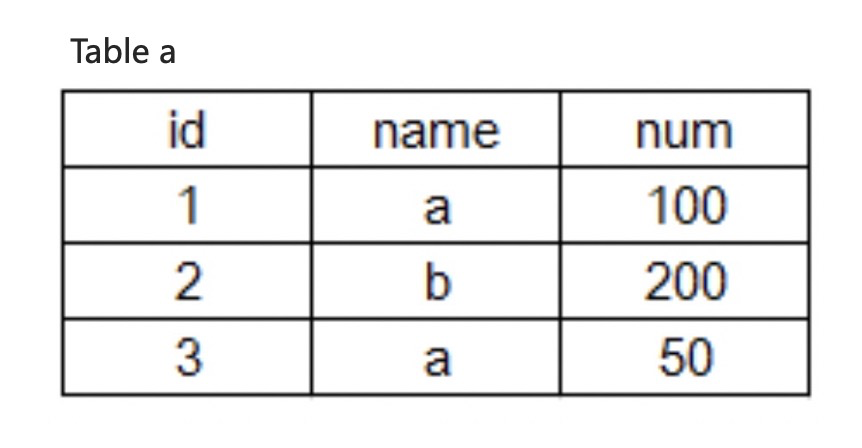• Query the name column from table a.

``SELECT name FROM a; ``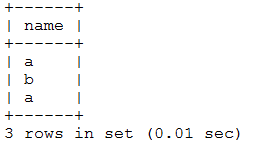• Return the distinct rows for the name column.

``SELECT DISTINCT name FROM a;``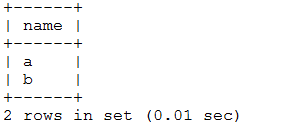• In table a, query the id, name, and num columns, divide the num column values by 2, and return the calculation result of the num column in a column named avg.

``SELECT id, name, num/2 AS avg FROM a;``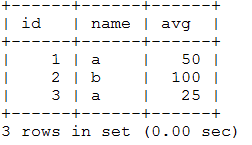• In table a, find the 'a' value for the name column, and return the values for the id, name, and num columns.

``SELECT id, name, num FROM a WHERE name = 'a';``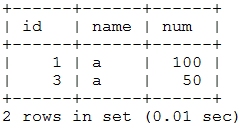• In table a, query the id and name columns, group the num column values by name, and return the sum of the num column values.

``SELECT id, name, SUM(num) FROM a GROUP BY name;``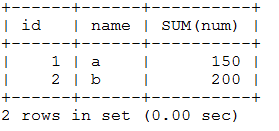• In table a, query the id and name columns, group the num column values by name, and calculate the sum of the num column values. Then, return the sum value that is less than 160.

``SELECT id, name, SUM(num) as sum FROM a GROUP BY name HAVING SUM(num) < 160;``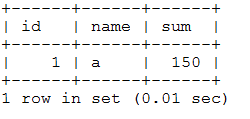• In table a, query the id, name, and num columns, and sort and return the result set by the num column in ascending order.

``SELECT * FROM a ORDER BY num ASC;``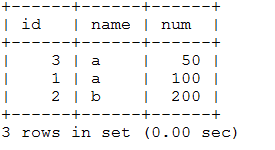• In table a, query the id, name, and num columns, and sort and return the result set by the num column in descending order.

``SELECT * FROM a ORDER BY num DESC;``• In table a, query the id, name, and num columns, and use the LIMIT clause to return two rows that start from the second row.

``SELECT * FROM a LIMIT 1,2;``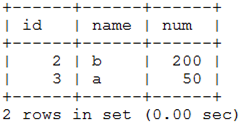## SELECT statements that contain set operators

### Description

You can execute the SELECT statement that contains the UNION, MINUS, or INTERSECT operator to combine query results.

### Syntax

``````select_clause_set:
simple_select [ UNION | UNION ALL | EXCEPT | INTERSECT] select_clause_set_left
[ORDER BY sort_list_columns] [limit_clause]
select_clause_set_right:
simple_select |
select_caluse_set``````

### Parameters

Parameter

Description

UNION ALL

Combine the results of two queries and return all rows.

UNION

Combine the results of two queries and return distinct rows.

EXCEPT

Return the distinct rows in the left query that are not selected by the right query.

INTERSECT

Return the distinct rows that are selected by the left and right queries.

### Examples

The following examples are based on tables t1 and t2.

``````create table t1 (c1 int, c2 int);
create table t2 (c1 int, c2 int);
insert into t1 values (1, -1), (2, -2);
insert into t2 values (1, 1), (2, -2), (3, 3);``````
• Query all rows from t1 and t2.

``````SELECT C1, C2 FROM T1 UNION ALL SELECT C1, C2 FROM T2;
+------+------+
| C1   | C2   |
+------+------+
|    1 |   -1 |
|    2 |   -2 |
|    1 |    1 |
|    2 |   -2 |
|    3 |    3 |
+------+------+``````
• Query all distinct rows from t1 and t2.

``````SELECT C1, C2 FROM T1 UNION SELECT C1, C2 FROM T2;
+------+------+
| C1   | C2   |
+------+------+
|    1 |   -1 |
|    2 |   -2 |
|    1 |    1 |
|    3 |    3 |
+------+------+``````
• Query the rows that exist in t1 and t2.

``````SELECT C1, C2 FROM T1 INTERSECT SELECT C1, C2 FROM T2;
+------+------+
| C1   | C2   |
+------+------+
|    2 |   -2 |
+------+------+``````
• Query the rows that exist in t1 but do not exist in t2.

``````SELECT C1, C2 FROM T1 EXCEPT SELECT C1, C2 FROM T2;
+------+------+
| C1   | C2   |
+------+------+
|    1 |   -1 |
+------+------+``````
• Query all the distinct rows from t1 and t2, and return the first two rows ordered by the c2 column in descending order.

``````SELECT C1, C2 FROM T1 UNION SELECT C1, C2 FROM T2 ORDER BY C2 DESC LIMIT 2;
+------+------+
| C1   | C2   |
+------+------+
|    3 |    3 |
|    1 |    1 |
+------+------+``````